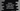# Python numpy logical AND example## How to do logical AND in numpy:

Logical and or AND can be done with the items of two arrays easily using numpy. numpy provides one method called logical_and that can be used for that.

In this post, I will show you how to use logical_and with examples.

### Definition of logical_and:

logical_and is defined as below:

``python.logical_and(arr1, arr2, out=None, where=True, dtype=None)``

Here,

• arr1 and arr2 are the given arrays. The must be of same shape. They must be broadcastable to a common shape if they are different in shape. The output array will be of same shape.
• out is the location where the final result is stored. It is an optional value. If not given or None, one newly allocated array is returned. It can be ndarray, None, or tuple of ndarray and None
• where is array_like value and it is optional. It is broadcasted over the array items. Where it is True, the array item will be set to the ufunc result, else it will take the original value.
• dtype is an optional value. It defines the type of the returned array.
• It returns a ndarray or a boolean value

### Example of logical_and:

Let’s start from a simple example. For the below example:

``````import numpy

print(numpy.logical_and(True, True))
print(numpy.logical_and(True, False))
print(numpy.logical_and(False, True))
print(numpy.logical_and(False, False))``````

It will print the below output:

``````True
False
False
False``````

### Example 2:

Let’s take an example of two arrays:

``````import numpy

arr1 = [True, False, False, True]
arr2 = [False, True, False, True]

print(numpy.logical_and(arr1, arr2))``````

It will print the below output:

``[False False False  True]``

### Example 3:

We can also use AND with numbers:

``````import numpy

arr1 = [1, 0, 0, 1]
arr2 = [0, 1, 0, 1]

print(numpy.logical_and(arr1, arr2))``````

It considers 0 as False and 1 as True. It will print the same output as the above example.

``[False False False  True]``

### Using where:

The below example shows how to use where:

``````import numpy

arr1 = [1, 0, 0, 1]
arr2 = [0, 1, 0, 1]

print(numpy.logical_and(arr1, arr2, where=[True, False, True, False]))``````

It will print:

``[False  True False  True]``